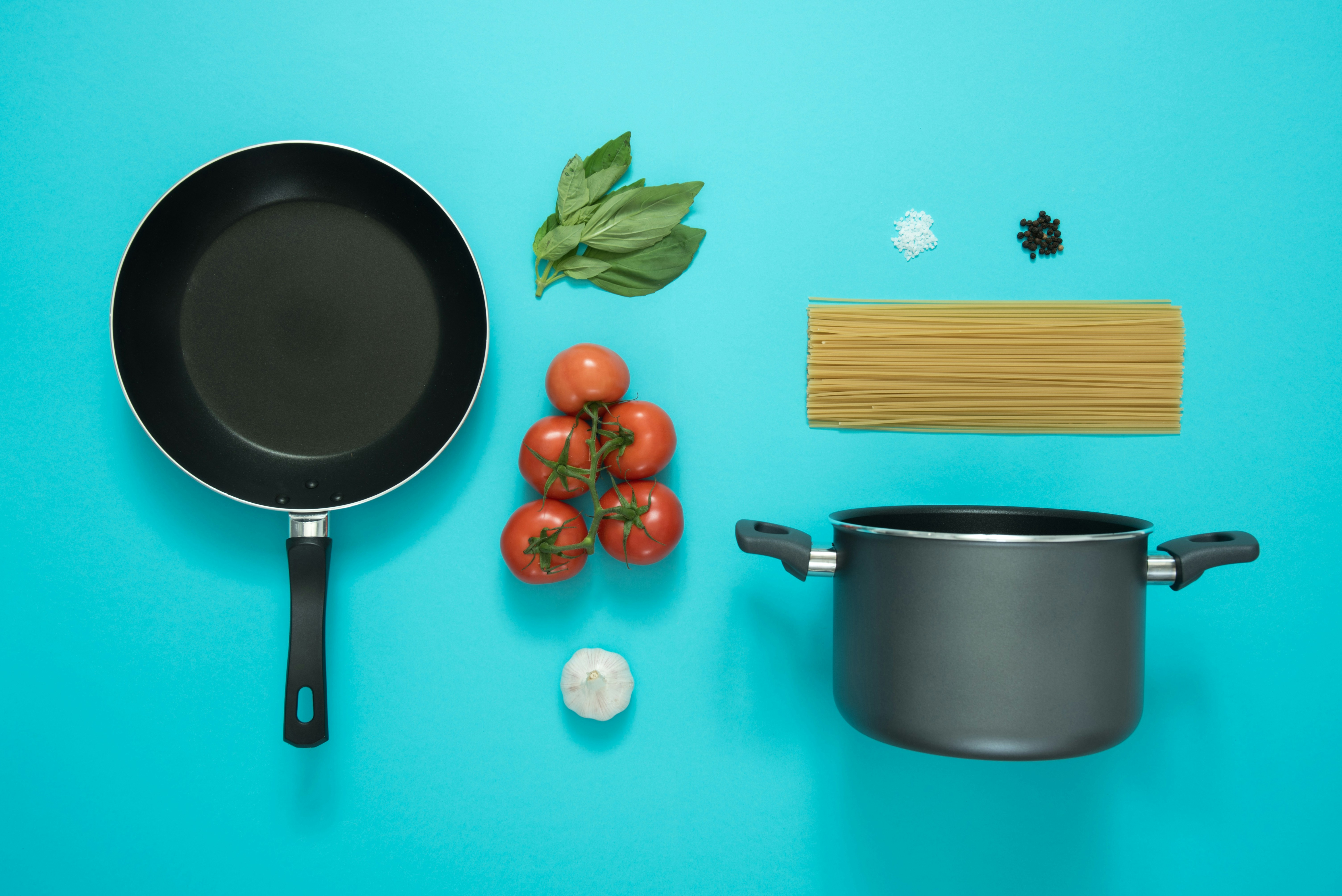Cooking Measurements & Conversion Chart
Share
ExploreFollow Coda's practical and easy-to-use conversion table for all your milliliters to cup conversion needs. You can use this conversion calculator for doing any metric conversions for cooking, baking or serving. Our conversion chart makes it easy to get accurate volume conversions for wet ingredients and more easily follow recipes that require measuring cups.

# Milliliters to Cups FAQs

Here are the answers to the most popular questions about milliliters to cups conversion.

### Is 250 milliliters about 1 cup?

By metric standards, 250 milliliters is considered 1 metric cup. However, by the US cup measure, 250 milliliters is equal to 1 cup and 1 tablespoon.

### How many milliliters is 1 US cup?

There is 236.588 millilitres in 1 standard US cup.

### Is 1 cup 200mL or 250mL?

This depends on whether your recipe is using the metric system or US cup measurement. 1 cup is 250ml in by metric standards.
`This cooking measurement tool is built in Coda. What is Coda⁠?`
Share